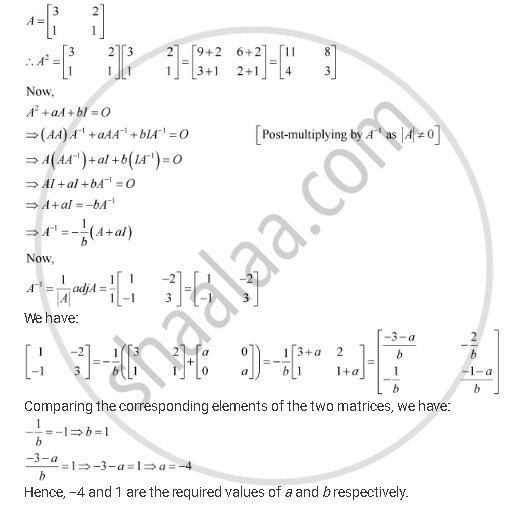Share

# For the Matrix a = [(3,2),(1,1)] Find the Numbers A And B Such That A2 + Aa + Bi = O. - CBSE (Science) Class 12 - Mathematics

#### Question

For the matrix A = [(3,2),(1,1)] find the numbers a and b such that A2 + aA + bI O.

#### SolutionIs there an error in this question or solution?

#### Video TutorialsVIEW ALL 

Solution For the Matrix a = [(3,2),(1,1)] Find the Numbers A And B Such That A2 + Aa + Bi = O. Concept: Adjoint and Inverse of a Matrix.
S